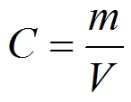PhysiologyWeb  Home  |  FAQ  |  About  |  Contact  |   - Sign InMass per Volume Solution Concentration Calculator
Meant to be used in both the teaching and research laboratory, this calculator (see below) can be utilized to perform a number of different calculations for preparing solutions having mass per volume (i.e., mass over volume) or weight per volume (i.e., weight over volume) concentration units such as mg/mL, μg/μL, μg/L, etc. Such concentration calculations are needed when starting with the solid form of a chemical and a solution needs to be prepared with the concentration unit expressed in unit mass per unit volume (such as mg/mL). These calculations are especially useful when working with compounds that do not have well-defined molecular weights (such as some proteins, carbohydrates, and other polymers).
If you wish to perform dilution calculations for solutions with mass per volume or weight per volume concentration units (e.g., mg/mL, μg/μL, μg/L, etc.), use our Dilution Calculator - Mass per Volume.
If you wish to perform dilution factor or fold dilution calculations for solutions with mass per volume or weight per volume concentration units (e.g., mg/mL, μg/μL, μg/L, etc.), use our Dilution Factor Calculator - Mass per Volume.
Mass per volume (mass / volume) concentration equation• C is the desired concentration of the final solution with the concentration unit expressed in units of mass per volume of solution (e.g., mg/mL).
• m is the mass (i.e., weight) of solute that must be dissolved in volume V of solution to make the desired solution concentration (C).
• V is volume of solution in which the indicated mass (m) of solute must be dissolved to make the desired solution concentration (C). Note that V is the final or total volume of solution after the solute has been added to the solvent.
Mass per volume (mass / volume) solution concentration calculator
Each calculator cell shown below corresponds to a term in the formula presented above. Enter appropriate values in all cells except the one you wish to calculate. Therefore, at least two cells must have values, and no more than one cell may be blank. The value of the blank cell will be calculated based on the other values entered. After a calculation is performed, the calculated cell will be highlighted and subsequent calculations will calculate the value of the highlighted cell (with no requirement to have a blank cell). However, a blank cell has priority over a highlighted cell.
For convenience, this calculator allows you to select different mass, volume, and concentration units, and the necessary conversions are carried out for you to obtain the value of the blank cell in the desired unit.
 Mass of solute g pg ng μg mg g kg Total solution volume mL fL pL nL μL mL L Concentration (mass per volume) mg/mL pg ng μg mg g kg per fL pL nL μL mL L